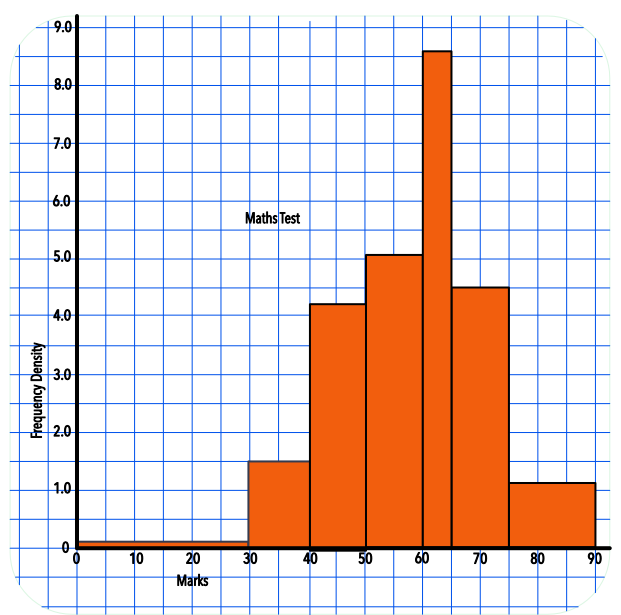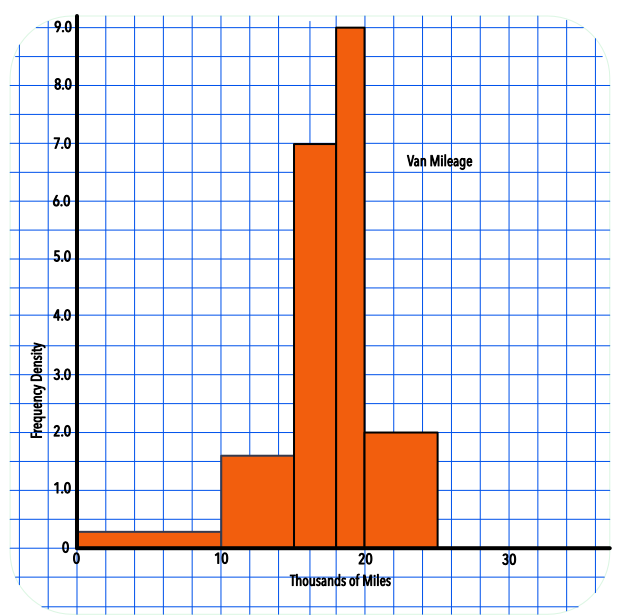Histograms with Unequal Intervals

# Histograms with Unequal Intervals

GCSE(H),

For histograms, the heights of the bars on a histogram indicate the Frequency Density. The area of each bar is the frequency. The Frequency Density changes for each bar on the histogram:

Frequency Density = frac(text(frequency))(text(class width))

The example shows a table of a maths test given to a year group. Note that the class interval varies for each class:

HourFrequencyClass widthFrequency Density
0 < h ≤ 30 2 302 ÷ 30 = 0.1
30 < h ≤ 40 181018 ÷ 10 = 1.5
40 < h ≤ 50 421042 ÷ 10 = 4.2
50 < h ≤ 60 511051 ÷ 10 = 5.1
60 < h ≤ 65 43 543 ÷ 5 = 8.6
65 < h ≤ 75 451045 ÷ 10 = 4.5
75 < h ≤ 90 181518 ÷ 15 = 1.2## Examples

1. A survey was made on the mileage covered by vans in a delivery fleet. The bottom scale shows thousands of miles. Using the histogram, determine how many vans completed between 10000 and 15000 miles.The class interval is 5.

The frequency density is 1.6.

Frequency = class interval x frequency density

5 x 1.6 = 8

2. The value for mileage between 25000 and 32000 miles has not been plotted. If the number of vans was 7, and the scale is showing thousands of miles, what height should the bar be?

Frequency density = frac(text(frequency))(text(interval)) = frac(7)(7) = 1